Electromagnetic radiation (often abbreviated E-M radiation or EMR) is a form of energy that exhibits wave-like behavior as it travels through space. EMR has both electric and magnetic field components, which oscillate in phase perpendicular to each other and perpendicular to the direction of energy propagation.

Electromagnetic radiation is classified according to the frequency of its wave. The electromagnetic spectrum, in order of increasing frequency and decreasing wavelength, consists of radio waves, microwaves, infrared radiation, visible light, ultraviolet radiation, X-rays and gamma rays. The eyes of various organisms sense a small and somewhat variable window of frequencies called the visible spectrum. The photon is the quantum of the electromagnetic interaction and the basic "unit" of light and all other forms of electromagnetic radiation and is also the force carrier for the electromagnetic force.

Electromagnetic radiation carries energy - sometimes called radiant energy - and momentum that may be imparted to matter with which it interacts, through absorption of electromagnetic radiation.

## Physics

### Theory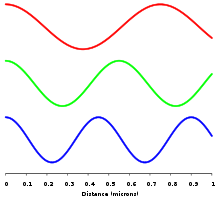Shows the relative wavelengths of the electromagnetic waves of three different colors of light (blue, green, and red) with a distance scale in micrometres along the x-axis.

James Clerk Maxwell first formally postulated electromagnetic waves. These were subsequently confirmed by Heinrich Hertz. Maxwell derived a wave form of the electric and magnetic equations, thus uncovering the wave-like nature of electric and magnetic fields, and their symmetry. Because the speed of EM waves predicted by the wave equation coincided with the measured speed of light, Maxwell concluded that light itself is an EM wave.

According to Maxwell's equations, a spatially varying electric field causes the magnetic field to change over time. Likewise, a spatially varying magnetic field causes changes over time in the electric field. In an electromagnetic wave, the changes induced by the electric field shift the wave in the magnetic field in one direction; the action of the magnetic field shifts the electric field in the same direction. Together, these fields form a propagating electromagnetic wave. This view of propagating electromagnetic waves makes sense from a local perspective, but note that some prefer instead to look into the past for the source charge(s) that were the original cause of the wave.

A quantum theory of the interaction between electromagnetic radiation and matter such as electrons is described by the theory of quantum electrodynamics.

### Properties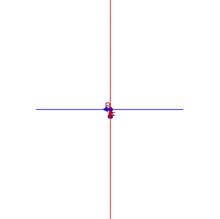The electric field is in a vertical plane and the magnetic field in a horizontal plane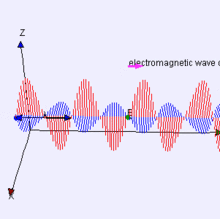Electromagnetic waves can be imagined as a self-propagating transverse oscillating wave of electric and magnetic fields. This 3D diagram shows a plane linearly polarized wave propagating from left to right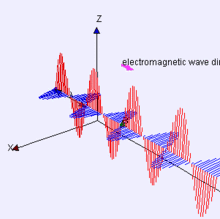This 3D diagram shows a plane linearly polarized wave propagating from left to right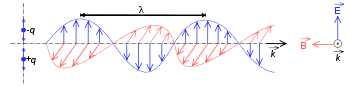Electromagnetic waves can be imagined as a self-propagating transverse oscillating wave of electric and magnetic fields. This diagram shows a plane linearly polarized wave propagating from left to right. The electric field is in a vertical plane and the magnetic field in a horizontal plane.

The physics of electromagnetic radiation is electrodynamics. Electromagnetism is the physical phenomenon associated with the theory of electrodynamics. Electric and magnetic fields obey the properties of superposition. Thus, a field due to any particular particle or time-varying electric or magnetic field contributes to the fields present in the same space due to other causes. Further, as they are vector fields, all magnetic and electric field vectors add together according to vector addition. For example, in optics two or more coherent lightwaves may interact and by constructive or destructive interference yield a resultant irradiance deviating from the sum of the component irradiances of the individual lightwaves.

Since light is an oscillation it is not affected by travelling through static electric or magnetic fields in a linear medium such as a vacuum. However in nonlinear media, such as some crystals, interactions can occur between light and static electric and magnetic fields — these interactions include the Faraday effect and the Kerr effect.

In refraction, a wave crossing from one medium to another of different density alters its speed and direction upon entering the new medium. The ratio of the refractive indices of the media determines the degree of refraction, and is summarized by Snell's law. Light of composite wavelengths (natural sunlight) disperses into a visible spectrum passing through a prism, because of the wavelength dependent refractive index of the prism material (dispersion); that is, each component wave within the composite light is bent a different amount.

EM radiation exhibits both wave properties and particle properties at the same time (see wave-particle duality). Both wave and particle characteristics have been confirmed in a large number of experiments. Wave characteristics are more apparent when EM radiation is measured over relatively large timescales and over large distances while particle characteristics are more evident when measuring small timescales and distances. For example, when electromagnetic radiation is absorbed by matter, particle-like properties will be more obvious when the average number of photons in the cube of the relevant wavelength is much smaller than 1. Upon absorption of light, it is not too difficult to experimentally observe non-uniform deposition of energy. However, this alone is not evidence of "particulate" behavior of light. Rather, it reflects the quantum nature of matter.

There are experiments in which the wave and particle natures of electromagnetic waves appear in the same experiment, such as the self-interference of a single photon. True single-photon experiments (in a quantum optical sense) can be done today in undergraduate-level labs. When a single photon is sent through an interferometer, it passes through both paths, interfering with itself, as waves do, yet is detected by a photomultiplier or other sensitive detector only once.

### Wave model

Electromagnetic radiation is a transverse wave meaning that the oscillations of the waves are perpendicular to the direction of energy transfer and travel. An important aspect of the nature of light is frequency. The frequency of a wave is its rate of oscillation and is measured in hertz, the SI unit of frequency, where one hertz is equal to one oscillation per second. Light usually has a spectrum of frequencies that sum to form the resultant wave. Different frequencies undergo different angles of refraction.

A wave consists of successive troughs and crests, and the distance between two adjacent crests or troughs is called the wavelength. Waves of the electromagnetic spectrum vary in size, from very long radio waves the size of buildings to very short gamma rays smaller than atom nuclei. Frequency is inversely proportional to wavelength, according to the equation:$\displaystyle v=f\lambda$

where v is the speed of the wave (c in a vacuum, or less in other media), f is the frequency and λ is the wavelength. As waves cross boundaries between different media, their speeds change but their frequencies remain constant.

Interference is the superposition of two or more waves resulting in a new wave pattern. If the fields have components in the same direction, they constructively interfere, while opposite directions cause destructive interference.

The energy in electromagnetic waves is sometimes called radiant energy.

### Particle model

Because energy of an electromagnetic wave is quantized (see second quantization), electromagnetic energy is emitted and absorbed as discrete packets of energy, or quanta, called photons. The energy of the photons is proportional to the frequency of the wave. On the converse, in a first-quantized treatment, because a photon acts as a transporter of energy, it is associated with a probability wave with frequency proportional to the energy carried. In both treatments, the energy per photon is related to the frequency via the Planck–Einstein equation:$\displaystyle E=hf$

where E is the energy, h is Planck's constant, and f is frequency. The energy is commonly expressed in the unit of electronvolt (eV). This photon-energy expression is a particular case of the energy levels of the more general electromagnetic oscillator, whose average energy, which is used to obtain Planck's radiation law, can be shown to differ sharply from that predicted by the equipartition principle at low temperature, thereby establishes a failure of equipartition due to quantum effects at low temperature.

As a photon is absorbed by an atom, it excites the atom, elevating an electron to a higher energy level. If the energy is great enough, so that the electron jumps to a high enough energy level, it may escape the positive pull of the nucleus and be liberated from the atom in a process called photoionisation. However, an electron that descends to a lower energy level in an atom emits a photon of light equal to the energy difference. Since the energy levels of electrons in atoms are discrete, each element emits and absorbs its own characteristic frequencies.

Together, these effects explain the emission and absorption spectra of light. The dark bands in the absorption spectrum are due to the atoms in the intervening medium absorbing different frequencies of the light. The composition of the medium through which the light travels determines the nature of the absorption spectrum. For instance, dark bands in the light emitted by a distant star are due to the atoms in the star's atmosphere. These bands correspond to the allowed energy levels in the atoms. A similar phenomenon occurs for emission. As the electrons descend to lower energy levels, a spectrum is emitted that represents the jumps between the energy levels of the electrons. This is manifested in the emission spectrum of nebulae. Today, scientists use this phenomenon to observe what elements a certain star is composed of. It is also used in the determination of the distance of a star, using the red shift.

### Speed of propagation

Any electric charge that accelerates, or any changing magnetic field, produces electromagnetic radiation. Electromagnetic information about the charge travels at the speed of light. Accurate treatment thus incorporates a concept known as retarded time (as opposed to advanced time, which is not physically possible in light of causality), which adds to the expressions for the electrodynamic electric field and magnetic field. These extra terms are responsible for electromagnetic radiation. When any wire (or other conducting object such as an antenna) conducts alternating current, electromagnetic radiation is propagated at the same frequency as the electric current. At the quantum level, electromagnetic radiation is produced when the wavepacket of a charged particle oscillates or otherwise accelerates. Charged particles in a stationary state do not move, but a superposition of such states may result in oscillation, which is responsible for the phenomenon of radiative transition between quantum states of a charged particle.

Depending on the circumstances, electromagnetic radiation may behave as a wave or as particles. As a wave, it is characterized by a velocity (the speed of light), wavelength, and frequency. When considered as particles, they are known as photons, and each has an energy related to the frequency of the wave given by Planck's relation E = hν, where E is the energy of the photon, h = 6.626 × 10−34 J·s is Planck's constant, and ν is the frequency of the wave.

One rule is always obeyed regardless of the circumstances: EM radiation in a vacuum always travels at the speed of light, relative to the observer, regardless of the observer's velocity. (This observation led to Albert Einstein's development of the theory of special relativity.)

In a medium (other than vacuum), velocity factor or refractive index are considered, depending on frequency and application. Both of these are ratios of the speed in a medium to speed in a vacuum.

The inverse or time-reversed process of absorption is responsible for thermal radiation. Much of the thermal energy in matter consists of random motion of charged particles, and this energy can be radiated away from the matter. The resulting radiation may subsequently be absorbed by another piece of matter, with the deposited energy heating the material. Radiation is an important mechanism of heat transfer.

The electromagnetic radiation in an opaque cavity at thermal equilibrium is effectively a form of thermal energy, having maximum radiation entropy. The thermodynamic potentials of electromagnetic radiation can be well-defined as for matter. Thermal radiation in a cavity has energy density (see Planck's Law) of${U\over V} = \frac{8\pi^5(kT)^4}{15 (hc)^3},$

Differentiating the above with respect to temperature, we may say that the electromagnetic radiation field has an effective volumetric heat capacity given by$\frac{32\pi^5 k^4 T^3}{15 (hc)^3},$

## Electromagnetic spectrum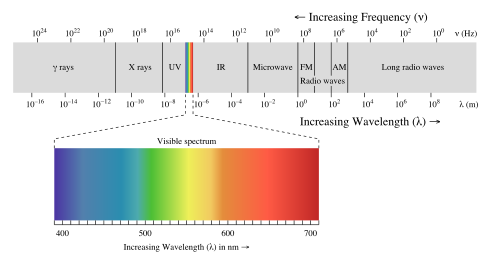Electromagnetic spectrum with light highlighted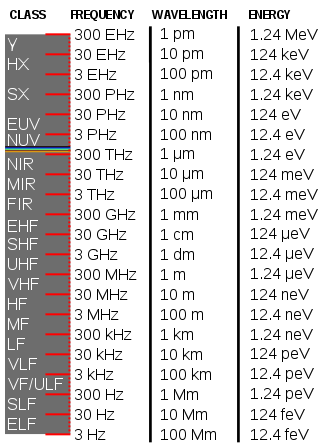Legend:
γ = Gamma rays
HX = Hard X-rays
SX = Soft X-Rays
EUV = Extreme-ultraviolet
NUV = Near-ultraviolet
Visible light
NIR = Near-infrared
MIR = Moderate-infrared
FIR = Far-infrared

EHF = Extremely high frequency (Microwaves)
SHF = Super-high frequency (Microwaves)
UHF = Ultrahigh frequency
VHF = Very high frequency
HF = High frequency
MF = Medium frequency
LF = Low frequency
VLF = Very low frequency
VF = Voice frequency
ULF = Ultra-low frequency
SLF = Super-low frequency
ELF = Extremely low frequency

In general, EM radiation (the designation 'radiation' excludes static electric and magnetic and near fields) is classified by wavelength into radio, microwave, infrared, the visible region we perceive as light, ultraviolet, X-rays, and gamma rays. Arbitrary electromagnetic waves can always be expressed by Fourier analysis in terms of sinusoidal monochromatic waves, which can be classified into these regions of the spectrum.

The behavior of EM radiation depends on its wavelength. Higher frequencies have shorter wavelengths, and lower frequencies have longer wavelengths. When EM radiation interacts with single atoms and molecules, its behavior depends on the amount of energy per quantum it carries. Spectroscopy can detect a much wider region of the EM spectrum than the visible range of 400 nm to 700 nm. A common laboratory spectroscope can detect wavelengths from 2 nm to 2500 nm. Detailed information about the physical properties of objects, gases, or even stars can be obtained from this type of device. It is widely used in astrophysics. For example, hydrogen atoms emit radio waves of wavelength 21.12 cm.

Soundwaves are not electromagnetic radiation. At the lower end of the electromagnetic spectrum, about 20 Hz to about 20 kHz, are frequencies that might be considered in the audio range. However, electromagnetic waves cannot be directly perceived by human ears. Sound waves are the oscillating compression of molecules. To be heard, electromagnetic radiation must be converted to air pressure waves, or if the ear is submerged, water pressure waves.

### Light

EM radiation with a wavelength between approximately 400 nm and 700 nm is directly detected by the human eye and perceived as visible light. Other wavelengths, especially nearby infrared (longer than 700 nm) and ultraviolet (shorter than 400 nm) are also sometimes referred to as light, especially when visibility to humans is not relevant.

If radiation having a frequency in the visible region of the EM spectrum reflects off of an object, say, a bowl of fruit, and then strikes our eyes, this results in our visual perception of the scene. Our brain's visual system processes the multitude of reflected frequencies into different shades and hues, and through this not-entirely-understood psychophysical phenomenon, most people perceive a bowl of fruit.

At most wavelengths, however, the information carried by electromagnetic radiation is not directly detected by human senses. Natural sources produce EM radiation across the spectrum, and our technology can also manipulate a broad range of wavelengths. Optical fiber transmits light, which, although not suitable for direct viewing, can carry data that can be translated into sound or an image. To be meaningful both transmitter and receiver must use some agreed-upon encoding system - especially so if the transmission is digital as opposed to the analog nature of the waves.

Radio waves can be made to carry information by varying the amplitude, frequency or phase.

When EM radiation impinges upon a conductor, it couples to the conductor, travels along it, and induces an electric current on the surface of that conductor by exciting the electrons of the conducting material. This effect (the skin effect) is used in antennas. EM radiation may also cause certain molecules to absorb energy and thus to heat up; this is exploited in microwave ovens. Radio waves are not ionizing radiation, as the energy per photon is too small.

## Derivation

Electromagnetic waves as a general phenomenon were predicted by the classical laws of electricity and magnetism, known as Maxwell's equations. Inspection of Maxwell's equations without sources (charges or currents) results in, along with the possibility of nothing happening, nontrivial solutions of changing electric and magnetic fields. Beginning with Maxwell's equations in free space:$\nabla \cdot \mathbf{E} = 0 \qquad \qquad \qquad \ \ (1)$$\nabla \times \mathbf{E} = -\frac{\partial \mathbf{B}}{\partial t} \qquad \qquad \ (2)$$\nabla \cdot \mathbf{B} = 0 \qquad \qquad \qquad \ \ (3)$$\nabla \times \mathbf{B} = \mu_0 \epsilon_0 \frac{\partial \mathbf{E}}{\partial t} \qquad \quad \ (4)$
where$\nabla$ is a vector differential operator (see Del).

One solution,$\mathbf{E}=\mathbf{B}=\mathbf{0},$

is trivial.

For a more useful solution, we utilize vector identities, which work for any vector, as follows:$\nabla \times \left( \nabla \times \mathbf{A} \right) = \nabla \left( \nabla \cdot \mathbf{A} \right) - \nabla^2 \mathbf{A}$

To see how we can use this, take the curl of equation (2):$\nabla \times \left(\nabla \times \mathbf{E} \right) = \nabla \times \left(-\frac{\partial \mathbf{B}}{\partial t} \right) \qquad \qquad \qquad \quad \ \ \ (5) \,$

Evaluating the left hand side:$\nabla \times \left(\nabla \times \mathbf{E} \right) = \nabla\left(\nabla \cdot \mathbf{E} \right) - \nabla^2 \mathbf{E} = - \nabla^2 \mathbf{E} \qquad \ \ (6) \,$
where we simplified the above by using equation (1).

Evaluate the right hand side:$\nabla \times \left(-\frac{\partial \mathbf{B}}{\partial t} \right) = -\frac{\partial}{\partial t} \left( \nabla \times \mathbf{B} \right) = -\mu_0 \epsilon_0 \frac{\partial^2 \mathbf{E}}{\partial t^2} \quad \ \ \ \ (7)$

Equations (6) and (7) are equal, so this results in a vector-valued differential equation for the electric field, namely$\nabla^2 \mathbf{E} = \mu_0 \epsilon_0 \frac{\partial^2 \mathbf{E}}{\partial t^2}$

Applying a similar pattern results in similar differential equation for the magnetic field:$\nabla^2 \mathbf{B} = \mu_0 \epsilon_0 \frac{\partial^2 \mathbf{B}}{\partial t^2}.$

These differential equations are equivalent to the wave equation:$\nabla^2 f = \frac{1}{{c_0}^2} \frac{\partial^2 f}{\partial t^2} \,$
where
c0 is the speed of the wave in free space and
f describes a displacement

Or more simply:$\Box f = 0$
where$\Box$ is d'Alembertian:$\Box = \nabla^2 - \frac{1}{{c_0}^2} \frac{\partial^2}{\partial t^2} = \frac{\partial^2}{\partial x^2} + \frac{\partial^2}{\partial y^2} + \frac{\partial^2}{\partial z^2} - \frac{1}{{c_0}^2} \frac{\partial^2}{\partial t^2} \$

Notice that, in the case of the electric and magnetic fields, the speed is:$c_0 = \frac{1}{\sqrt{\mu_0 \epsilon_0}}$

This is the speed of light in vacuum. Maxwell's equations have unified the vacuum permittivity$\epsilon_0$, the vacuum permeability μ0, and the speed of light itself, c0. Before this derivation it was not known that there was such a strong relationship between light and electricity and magnetism.

But these are only two equations and we started with four, so there is still more information pertaining to these waves hidden within Maxwell's equations. Let's consider a generic vector wave for the electric field.$\mathbf{E} = \mathbf{E}_0 f\left( \hat{\mathbf{k}} \cdot \mathbf{x} - c_0 t \right)$

Here,$\mathbf{E}_0$ is the constant amplitude, f is any second differentiable function,$\hat{\mathbf{k}}$ is a unit vector in the direction of propagation, and${\mathbf{x}}$is a position vector. We observe that$f\left( \hat{\mathbf{k}} \cdot \mathbf{x} - c_0 t \right)$ is a generic solution to the wave equation. In other words$\nabla^2 f\left( \hat{\mathbf{k}} \cdot \mathbf{x} - c_0 t \right) = \frac{1}{{c_0}^2} \frac{\partial^2}{\partial t^2} f\left( \hat{\mathbf{k}} \cdot \mathbf{x} - c_0 t \right),$

for a generic wave traveling in the$\hat{\mathbf{k}}$ direction.

This form will satisfy the wave equation, but will it satisfy all of Maxwell's equations, and with what corresponding magnetic field?$\nabla \cdot \mathbf{E} = \hat{\mathbf{k}} \cdot \mathbf{E}_0 f'\left( \hat{\mathbf{k}} \cdot \mathbf{x} - c_0 t \right) = 0$$\mathbf{E} \cdot \hat{\mathbf{k}} = 0$

The first of Maxwell's equations implies that electric field is orthogonal to the direction the wave propagates.$\nabla \times \mathbf{E} = \hat{\mathbf{k}} \times \mathbf{E}_0 f'\left( \hat{\mathbf{k}} \cdot \mathbf{x} - c_0 t \right) = -\frac{\partial \mathbf{B}}{\partial t}$$\mathbf{B} = \frac{1}{c_0} \hat{\mathbf{k}} \times \mathbf{E}$

The second of Maxwell's equations yields the magnetic field. The remaining equations will be satisfied by this choice of$\mathbf{E},\mathbf{B}$.

Not only are the electric and magnetic field waves traveling at the speed of light but they have a special restricted orientation and proportional magnitudes, E0 = c0B0, which can be seen immediately from the Poynting vector. The electric field, magnetic field, and direction of wave propagation are all orthogonal, and the wave propagates in the same direction as$\mathbf{E} \times \mathbf{B}$.

From the viewpoint of an electromagnetic wave traveling forward, the electric field might be oscillating up and down, while the magnetic field oscillates right and left; but this picture can be rotated with the electric field oscillating right and left and the magnetic field oscillating down and up. This is a different solution that is traveling in the same direction. This arbitrariness in the orientation with respect to propagation direction is known as polarization. On a quantum level, it is described as photon polarization. The direction of the polarization is defined as the direction of the electric field.

More general forms of the second-order wave equations given above are available, allowing for both non-vacuum propagation media and sources. A great many competing derivations exist, all with varying levels of approximation and intended applications. One very general example is a form of the electric field equation, which was factorized into a pair of explicitly directional wave equations, and then efficiently reduced into a single uni-directional wave equation by means of a simple slow-evolution approximation.

Wikimedia Foundation. 2010.

Поможем решить контрольную работу

### Look at other dictionaries:

• electromagnetic radiation — n a series of electromagnetic waves * * * see under wave …   Medical dictionary

• electromagnetic radiation — ► NOUN ▪ a kind of radiation including visible light, radio waves, gamma rays, and X rays, in which electric and magnetic fields vary simultaneously …   English terms dictionary

• Electromagnetic Radiation —   [engl.], elektromagnetische Strahlung …   Universal-Lexikon

• electromagnetic radiation — Physics. radiation consisting of electromagnetic waves, including radio waves, infrared, visible light, ultraviolet, x rays, and gamma rays. [1950 55] * * * Energy propagated through free space or through a material medium in the form of… …   Universalium

• electromagnetic radiation —    The energy (in the form of magnetic and electric fields) given off by a vibrating charge (such as an electron). Every physical object in the universe gives off electromagnetic radiation of one type or another. The phenomenon of sight is due to …   Forensic science glossary

• electromagnetic radiation — elektromagnetinė spinduliuotė statusas Aprobuotas sritis karo inžinerija apibrėžtis Kintamojo elektromagnetinio lauko energija, sklindanti kaip elektromagnetinės bangos. atitikmenys: angl. electromagnetic radiation pranc. rayonnement… …   Lithuanian dictionary (lietuvių žodynas)

• electromagnetic radiation — elektromagnetinė spinduliuotė statusas T sritis Standartizacija ir metrologija apibrėžtis Elektromagnetinės bangos, kurias kuria įvairūs spinduoliai. atitikmenys: angl. electromagnetic radiation vok. elektromagnetische Strahlung, f rus.… …   Penkiakalbis aiškinamasis metrologijos terminų žodynas

• electromagnetic radiation — elektromagnetinė spinduliuotė statusas T sritis fizika atitikmenys: angl. electromagnetic radiation vok. elektromagnetische Strahlung, f rus. электромагнитное излучение, n pranc. rayonnement électromagnétique, m …   Fizikos terminų žodynas

• electromagnetic radiation — elektromagnetinė spinduliuotė statusas T sritis apsauga nuo naikinimo priemonių apibrėžtis Elektromagnetinių bangų skleidimas įgreitintais elektros krūviais (pvz., kintamąja srove). Biologinį EMS, ypač ultravioletinio, rentgeno ir gama dažnio… …   Apsaugos nuo naikinimo priemonių enciklopedinis žodynas